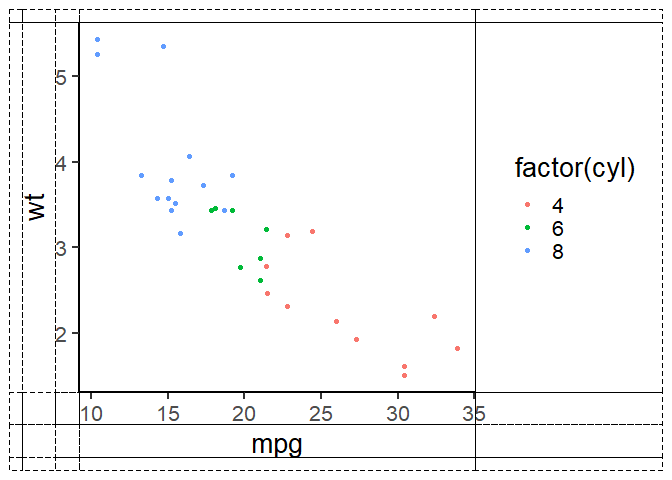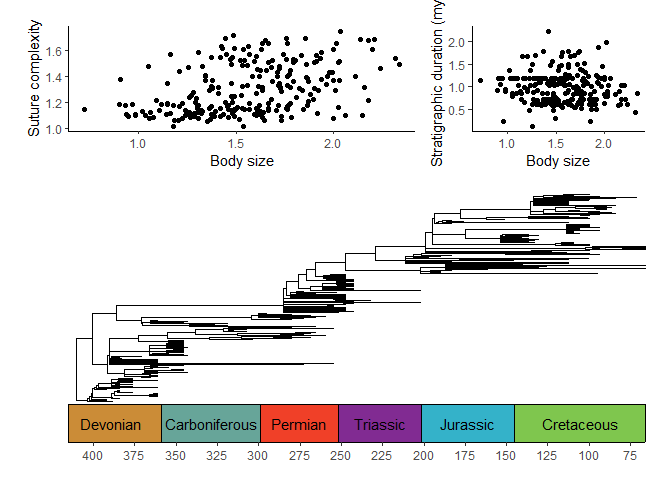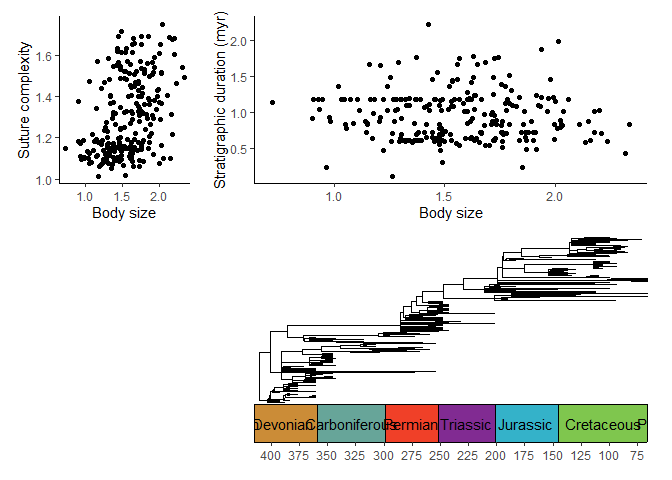# Combining and arranging plots

library(deeptime)
library(ggplot2)
library(ggtree)
# Load paleotree for some example data
library(paleotree)
data(RaiaCopesRule)

## The history of arranging and combining plots

Combining multiple plots together into figures is a key component of developing publishable data visualizations. Many other packages provide ways to combine and arrange plots. For example, the gridExtra package has the grid.arrange() function which allows the user to arrange plots in fairly complex layouts. However, the main drawback (for most cases) of this function is that the components of the plots (axes, labels, panels, etc.) are not aligned. The ggarrange() function in the egg package uses a 3x3 decomposition of the individual plots to align some of the plot components. The central cells correspond to the core panels, surrounded by axes, legends, etc.

## Arranging plots with deeptime

deeptime expands on this functionality with ggarrange2() to perform 7x7 decomposition of individual plots, as shown below. This ensures that more components of the individual plots are aligned with one another (e.g., the axis labels, the axis tick labels). The central cell corresponds to the plot panel(s), the rectangle of cells around that corresponds to the axes, the rectangle of cells around that corresponds to the axis titles, and the rectangle of cells around that corresponds to legends.

p1 <- ggplot(mtcars, aes(mpg, wt, colour = factor(cyl))) +
geom_point() +
theme_classic(base_size = 20)
ggarrange2(p1, debug = TRUE)## Combining plots

With this 7x7 decomposition process, it becomes very simple to combine plots into single figures with properly aligned components.

p1 <- ggplot(ammoniteTraitsRaia) +
geom_point(aes(x = Log_D, y = FD)) +
labs(x = "Body size", y = "Suture complexity") +
theme_classic()

p2 <- ggplot(ammoniteTraitsRaia) +
geom_point(aes(x = Log_D, y = log_dur)) +
labs(x = "Body size", y = "Stratigraphic duration (myr)") +
theme_classic()
gg1 <- ggarrange2(p1, p2, widths = c(2, 1), draw = FALSE)

You can chain calls to ggarrange2() to accomplish highly complex combinations and arrangements:

p3 <- ggtree(ammoniteTreeRaia, position = position_nudge(x = -ammoniteTreeRaia\$root.time)) +
coord_geo(
xlim = c(-415, -66), ylim = c(-2, Ntip(ammoniteTreeRaia)), pos = "bottom",
size = 4, abbrv = FALSE, neg = TRUE
) +
scale_x_continuous(breaks = seq(-425, -50, 25), labels = -seq(-425, -50, 25)) +
theme_tree2() +
theme(plot.margin = margin(7, 11, 7, 11))
ggarrange2(gg1, p3, nrow = 2, heights = c(1, 2))You can also accomplish complex plot layouts by supplying a matrix of integers to the layout argument, like with the base R layout() function. This can be used to insert empty plots or change the order of plots. Note that repeating a number will duplicate a plot, not expand it across multiple plot spots.

ggarrange2(p1, p2, p3,
layout = matrix(c(1, 2, 0, 3), nrow = 2, byrow = TRUE),
widths = c(1, 3)
)## Other resources for arranging plots

Note that I provide no guarantee that ggarrange2() solves every problem when arranging plots. The following other options may also be very useful:

• The {cowplot} package has the cowplot::plot_grid() function which accomplishes many of the same features as ggarrange2().
• The {patchwork} has very intuitive tools for combining and arranging plots (e.g, using mathematical symbols).